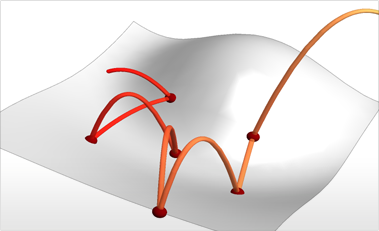# Advanced Hybrid and Differential Algebraic Equations

Mathematica 9 extends the broad language of modeling with differential equations to include advanced algorithms for solving differential-algebraic equations and hybrid systems with a mix of continuous- and discrete-time behavior. Automatic detection of discontinuous functions provides accurate solutions for discontinuous differential equations expressed in a natural mathematical specification.• Automatic detection and handling of discontinuous solutions.
• Filippov sliding mode solutions automatically computed when appropriate.
• New WhenEvent allows flexible specification of arbitrary events and corresponding actions.
• Discrete state changes expressed naturally through rules in WhenEvent.
• Variables that only change at discrete times can be added without increasing the differential system size and solving complexity.
• Advanced support for DAE allows for easier modeling of connected systems.
• Symbolic graph- and matrix-based index reduction methods for high-index DAEs.
• Advanced initialization algorithms for DAEs.
• New solving methods for DAEs.
• Arbitrary-precision solution of DAEs.

## Mathematica

Questions? Comments? Contact a Wolfram expert »